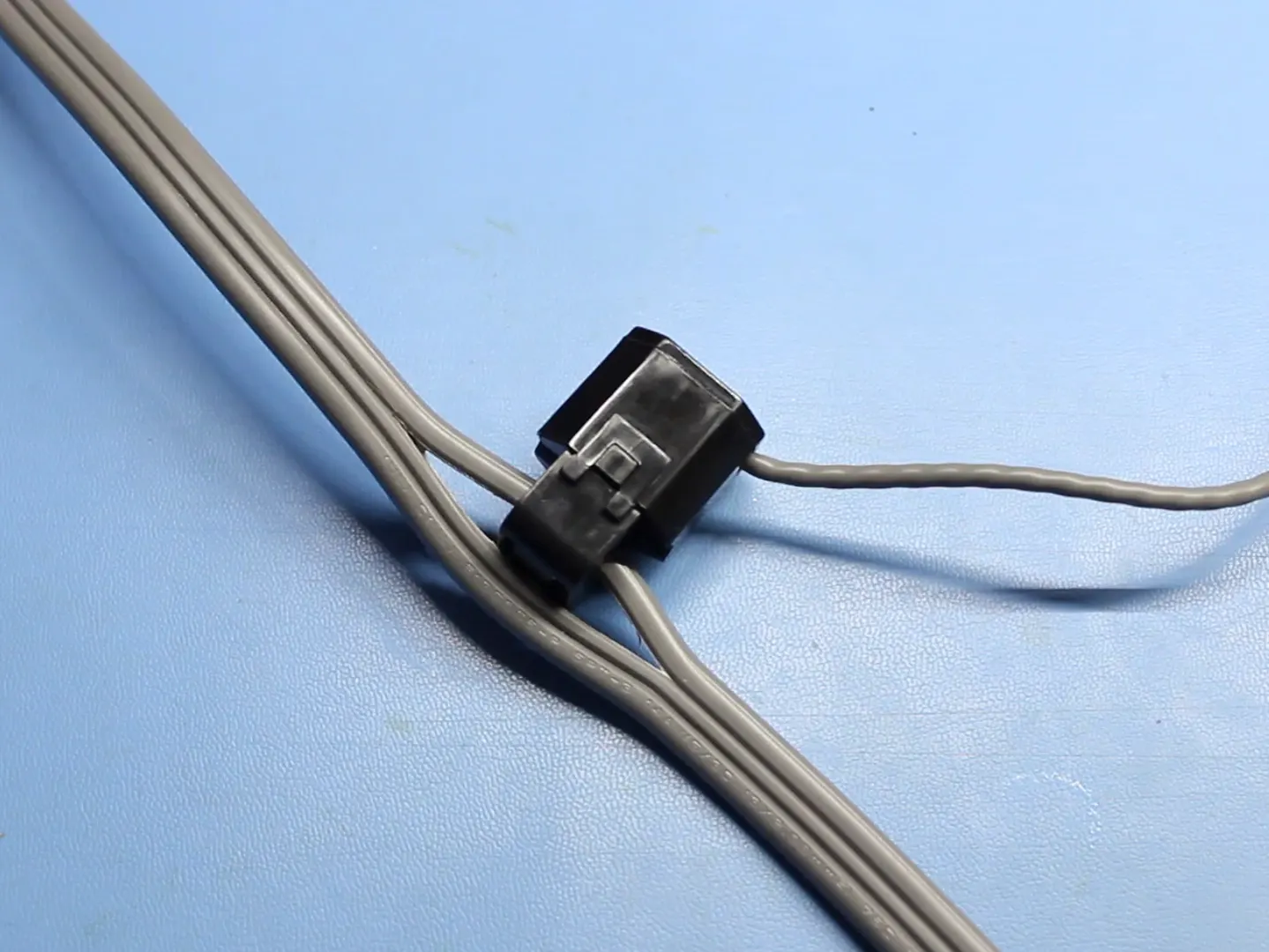# Hack Your Home Part 3: Power Monitor

Measure the current and power being used by an appliance and post that data to the Internet.

IntermediateFull instructions provided1 hour8,476## Things used in this project

### Hardware componentsParticle Photon
×1×1Jumper wires (generic)
×1
 USB microB Cable
×1
×1
 Audio Jack 3.5mm
×1
 Audio Jack Breakout
×1
×1
 Non-Invasive Current Sensor - 30A
×1
 Resistor 100 Ohm 1/4th Watt
×1
 Resistor 10k Ohm 1/6th Watt
×2
 Capacitor 10 μF
×1

### Hand tools and fabrication machines

 Hobby KnifeSoldering iron (generic)
 Solder

Fritzing diagram

## Code

### Power Monitor

Arduino
Copy this into a new app at build.particle.io
```#include "math.h"

// RMS voltage
const double vRMS = 120.0;      // Assumed or measured

// Parameters for measuring RMS current
const double offset = 1.65;     // Half the ADC max voltage
const int numTurns = 2000;      // 1:2000 transformer turns
const int rBurden = 100;        // Burden resistor value
const int numSamples = 1000;    // Number of samples before calculating RMS

void setup() {

}

void loop() {

int sample;
double voltage;
double iPrimary;
double acc = 0;
double iRMS;
double apparentPower;

// Take a number of samples and calculate RMS current
for ( int i = 0; i < numSamples; i++ ) {

voltage = (sample * 3.3) / 4096;
voltage = voltage - offset;

// Calculate the sensed current
iPrimary = (voltage / rBurden) * numTurns;

// Square current and add to accumulator
acc += pow(iPrimary, 2);
}

// Calculate RMS from accumulated values
iRMS = sqrt(acc / numSamples);

// Calculate apparent power and publish it
apparentPower = vRMS * iRMS;
Particle.publish("VA", String(apparentPower));

delay(10000);
}
```

## Credits

### Shawn hymel

10 projects • 112 followers
Engineering Superhero at SparkFun Electronics.